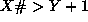Next: The fd Complex Constraints Up: The fd (Finite Domain) Previous: The fd Symbolic Finite

### The fd Integer Arithmetic Facilities

For numeric finite domains the fd library admits equations, inequalities and disequalities over numeric expressions.

Additionally the fd library includes some built-in optimisation predicates. These are all illustrated below.

```[eclipse 1]: lib(fd).
*       fd loaded

[eclipse 2]: X::1..10.
*       X = X{[1..10]}
*       yes.

[eclipse 3]: X::1..10, mindomain(X,Min).
*       X = X{[1..10]}
*       Min = 1
*       yes.

[eclipse 4]: [X,Y]::1..10, X#>Y+1.
*       X = X{[3..10]}
*       Y = Y{[1..8]}
*       yes.

[eclipse 5]: [X,Y]::1..10, X#>Y+1, Y#=6.
*       X = X{[8..10]}
*       Y = 6
*       yes.

[eclipse 6]: [X,Y,Z]::1..10, X #= 2*(Y+Z).
*       X = X{[4..10]}
*       Y = Y{[1..4]}
*       Z = Z{[1..4]}
*       yes.

[eclipse 7]: X::1..10, mindomain(X,Min).
*       X = X{[1..10]}
*       Min = 1
*       yes.

[eclipse 8]: [X,Y,Z]::1..10, X #= 2*(Y+Z), Y##Z,
minimize(labeling([X,Y,Z]),X).
*       Found a solution with cost 6
*       Y = 2
*       Z = 1
*       X = 6
*       yes.```
Numeric Finite Domains

Query 2 illustrates how a numeric finite domain can be initialised just by giving lower and upper bounds, instead of the whole list of members. In fact, internally, finite domains are stored as lists of intervals (for example [1..5, 8..10, 15]).

Query 3 shows how the user can find out the lower bound of a variable's numeric finite domain. There is a similar predicate for retrieving the upper bound.

Queries 4, 5 and 6 illustrate some features of finite domain constraint propagation.

Query 4 shows the pruning achieved by a simple numerical finite domain constraint. Notice that both the domains of X and Y are pruned - constraints work in all directions!

Query 5 illustrates that a finite domain constraint remains active even after it has achieved some pruning. This query is the same as query 3, with an extra constraint imposed subsequently. Theconstraint is still active, and prunes the domain of X still further from [3..10] to [8..10].

Query 6 shows that, in the interest of computational efficiency, the mathematical constraints only narrow the bounds of the finite domains. In this example the domain of X could theoretically be reduced to [4,6,8,10], but this would require much more computation - especially if the finite domains were quite large!

Query 7 is an example of the use of the built-in minimize predicate. This predicate returns an admissible labeling of the variables X, Y and Z which yields the smallest value for X. In general any search procedure can be substituted for labeling([X,Y,Z]) as the first argument to minimize. For example we could have used minimize( (indomain(X), indomain(Y), indomain(Z)), X).Next: The fd Complex Constraints Up: The fd (Finite Domain) Previous: The fd Symbolic Finite

Joachim Schimpf
Wed Sep 3 18:07:19 BST 1997